Wir verwenden Google für unsere Suche. Mit Klick auf „Suche aktivieren“ aktivieren Sie das Suchfeld und akzeptieren die Nutzungsbedingungen.

# New in SG-Lib 2.0

###### FLofTL(TL)- returns all facets of a tetrahedron list% FLofTL(TL) - returns all facets of a tetrahedron list% (by Tim Lueth, VLFL-Lib, 2014-DEZ-25 as class: TETRAHEDRONS)% % The nx4 list is converted into a (n*4)x3 list% FL=[TL(:,[2 3 4]); TL(:,[2 4 1]); TL(:,[4 3 1]); TL(:,[2 1 43])] % (Status of: 2014-12-26)% % FL=FLofTL(TL)% === INPUT PARAMETERS ===% TL: Tetrahedron list% === OUTPUT RESULTS ======% FL: Facet list (all normal vectors outside)%
###### ELorder(EL,jv)- reorders the edge list (triangle, tetrahedron) to individual polygons, surfaces, solids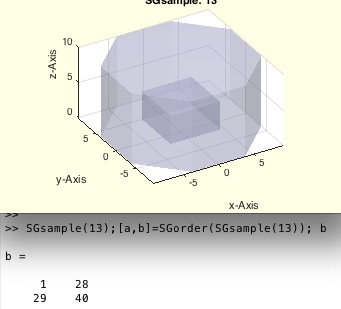% ELorder(EL,jv) - reorders the edge list (triangle, tetrahedron) to individual polygons, surfaces, solids% (by Tim Lueth, VLFL-Lib, 2014-DEZ-25 as class: AUXILIARY PROCEDURES)% % Equivalent to TRorder or SGorder but on edge level (in fact works with % all index lists). Improved in comparision with VLFLseparate.% All edges,facets,tetrahedrons of a solid use the same points. In case % that a solid geometry can be separated into several independent solids, % the given list here is ordered and in addition a list with starting % rows and end rows in the returned list is returned.% - For edges, one common point defines the attachment% - For facets, two common points define the attachment% - For tetrahedrons, three common points define the attachment% Anyway, exceptions are possible by the second input parameter% ELorder does not use the fnctn neighbors (Status of: 2017-02-21)% % See also: ELorder, FLorder, FLseparate, TRorder, SGorder, SGseparate% % [ELN,SIL]=ELorder(EL,[jv])% === INPUT PARAMETERS ===% EL: edge list/facet list/tetrahedron list consisting of several solids% jv: common vertices that define a connection; default is size(EL,2)-1% === OUTPUT RESULTS ======% ELN: ordered edge list/facet list/tetrahedron list of several solids% SIL: Solid index list (start and end-row) in returned list% % EXAMPLE: Separate the surface of SGsample(8) and SGsample(9)% SG=SGsample(8); % TR3=triangulation(SG.FL,SG.VL);% FEL=featureEdges(TR3,0.001); % [a,b]=ELorder(FEL); b%
###### exp_2014_12_25- EXPERIMENT to show different feature edges, facets, and tetrahedrons of a SGsample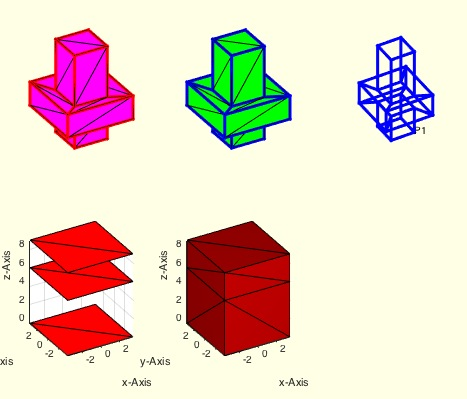% exp_2014_12_25 - EXPERIMENT to show different feature edges, facets, % and tetrahedrons of a SGsample% (by Tim Lueth, VLFL-Lib, 2014-DEZ-25 as class: EXPERIMENTS)% % exp_2014_12_25%
###### VLFLofTR(TR)- returns the freeboundary surface of a tetrahedron or the surface of a triangulation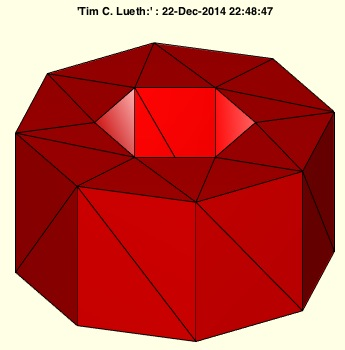% VLFLofTR(TR) - returns the freeboundary surface of a tetrahedron or the % surface of a triangulation% (by Tim Lueth, VLFL-Lib, 2014-DEZ-22 as class: TETRAHEDRONS)% % Returns the surface model (VL,FL) from a tetrahedron volume model by % using freeBoundary. If the triangulation is a surface model, the % ConnectivityList is returned. (Status of: 2014-12-22)% % [VL,FL]=VLFLofTR(TR)% === INPUT PARAMETERS ===% TR: Triangulation (nx4 or nx3)% === OUTPUT RESULTS ======% VL: Vertex List (Point List)% FL: Facet List (ConnectivityList or freeBoundary)% % EXAMPLE: % [VL,FL]=VLFLofTR(exp_2014_12_23 (SGsample(16))); close all; VLFLfigure; % view(-30,30); VLFLplots(VL,FL);%
###### exp_2014_12_23(SG)- EXPERIMENT returns a tetrahedron triangulation from a surface triangulation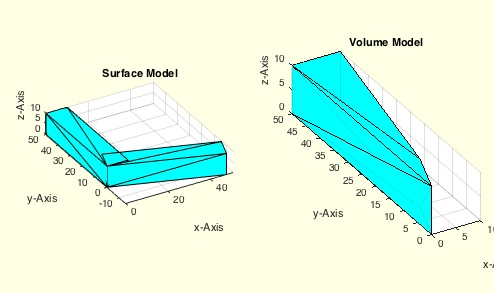% exp_2014_12_23(SG) - EXPERIMENT returns a tetrahedron triangulation % from a surface triangulation% (by Tim Lueth, VLFL-Lib, 2014-DEZ-22 as class: TETRAHEDRONS)% % This is the experiment to implement TRofSG (Status of: 2014-12-22)% % TR=exp_2014_12_23(SG)% === INPUT PARAMETERS ===% SG: Solid Geometry (VL,FL)% === OUTPUT RESULTS ======% TR: Tetrahedron triangulation%
###### exp_2014_12_22-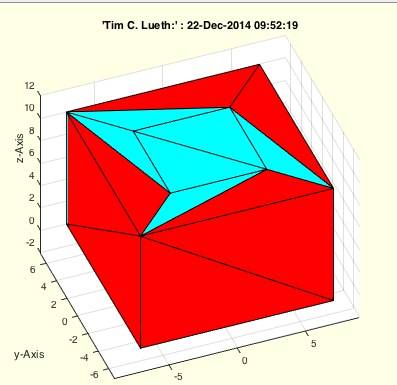% exp_2014_12_22 - % (by Tim Lueth, VLFL-Lib, 2014-DEZ-22 as class: EXPERIMENTS)% % exp_2014_12_22%
###### PLELofFeatureEdges(TR,alpha)- returns sorted closed polygon edge lists of the feature edges of a solid% PLELofFeatureEdges(TR,alpha) - returns sorted closed polygon edge lists of the feature edges of a solid% (by Tim Lueth, VLFL-Lib, 2014-DEZ-21 as class: SURFACES)% % fast and powerful fnctn that links the feature edges to closed polygon % edge lists with a defined normal vector% Uses: TRfeatureEdgeFacets (Status of: 2017-04-05)% % See also: FLfeatureEdgeSurface, FLfeatureEdgeSurface2, % PLELofFeatureEdges2, TRfeatureEdgeFacets, FEplot% % [ELFL,SIL]=PLELofFeatureEdges(TR,[alpha])% === INPUT PARAMETERS ===% TR: surface triangulation for feature edges% alpha: feature edge angle% === OUTPUT RESULTS ======% ELFL: % SIL: Selected Index List for % % EXAMPLE: Recreate the polygons of a % closeall; SG=SGsample(16); % TR=triangulation(SG.FL,SG.VL); % VLFLfigure; [ELFL,SIL]=PLELofFeatureEdges (TR);%
###### TRfeatureEdgeFacets(TR,alpha)- returns the list of facets linked to edges of the featureEdges% TRfeatureEdgeFacets(TR,alpha) - returns the list of facets linked to edges of the featureEdges% (by Tim Lueth, VLFL-Lib, 2014-DEZ-21 as class: SURFACES)% % EdgelistFacetlist% [edgestart (1) edgeend (2) facetattached (3) normalvectoroffacet (4:6)]% Quite frustrating. This fnctn took 30 minutes after 40 minutes sport % after several days unsuccessful programming exp_2014_12_16. (Status of: % 2017-04-05)% % See also: FEplot, FLfeatureEdgeSurface, FLfeatureEdgeSurface2, % PLELofFeatureEdges, PLELofFeatureEdges2, FEplot% % [ELFL]=TRfeatureEdgeFacets(TR,[alpha])% === INPUT PARAMETERS ===% TR: triangulation nx3% alpha: filterangle for featureEdges% === OUTPUT RESULTS ======% [ELFL]: %
###### SGorder(SG)- reorders the triangle facets to individual solids% SGorder(SG) - reorders the triangle facets to individual solids% (by Tim Lueth, VLFL-Lib, 2014-DEZ-20 as class: AUXILIARY PROCEDURES)% % Equivalent to TRorder but on facet level (one day may be fused). % Improved in comparision with VLFLseparate.% % All surface facets of a solid use the same points. In case that a solid % geometry has a facet list that in fact is separated into several % solids, this fnctns reorders the facet list to connected surfaces and % returns also a list with starting facet and end facet of the list. % (Status of: 2017-02-21)% % See also: ELorder, FLorder, FLseparate, TRorder, SGseparate% % [SG,SIL]=SGorder(SG)% === INPUT PARAMETERS ===% SG: Solid geometry whose facet list consisting of several solids% === OUTPUT RESULTS ======% SG: Solid geometry whose facet list consisting of several solids% SIL: Solid index list (start and end-facet) in facet list% % EXAMPLE: Separate the surface of SGsample(13)% SGsample(13);[a,b]=SGorder(SGsample(13)); b%
###### ELselect(EL,i)- return all edges from an edge list start start or end with and index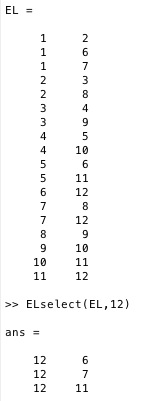% ELselect(EL,i) - return all edges from an edge list start start or end % with and index% (by Tim Lueth, VLFL-Lib, 2014-DEZ-19 as class: AUXILIARY PROCEDURES)% % fast efficient single row procedure (makro) (Status of: 2014-12-19)% % ELi=ELselect(EL,i)% === INPUT PARAMETERS ===% EL: Edge list% i: vertex index% === OUTPUT RESULTS ======% ELi: Edge list from index. ELi(:,1) is always i%
###### exp_2014_12_19 (SG,fa)- EXPERIMENT to create tetrahedrons from surfaces by using the feature-edges% exp_2014_12_19 (SG,fa) - EXPERIMENT to create tetrahedrons from % surfaces by using the feature-edges% (by Tim Lueth, VLFL-Lib, 2014-DEZ-19 as class: EXPERIMENTS)% % work in progress (Status of: 2014-12-22)% % exp_2014_12_19([SG,fa])% === INPUT PARAMETERS ===% SG: Solid geometry (surface model)% fa: feature edge angle%
###### exp_2014_12_16- PUBLISHABLE EXPERIMENT to understand the power of featureEdges for the reconstruction of solids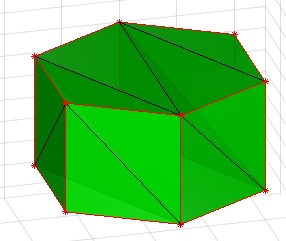% exp_2014_12_16 - PUBLISHABLE EXPERIMENT to understand the power of % featureEdges for the reconstruction of solids% (by Tim Lueth, VLFL-Lib, 2014-DEZ-16 as class: EXPERIMENTS)% % 1. This experiment starts with the construction of a surface model of a % n-edge polygon, extruded to a n-edge cylinder. It consists of a vertex % list and a surface facet list (VL,FL).% 2. Next we create a tetrahedron delaunay-triangulation based on the 3D % vertex list (TR). The facets created here does not have to be the same % as of the ones of the first surface generation. Could be but not have % to.% 3. We take the feature edge list which as to be the same for both % representations. Even if the facets were different since there is no % unique/bijective representation of a surface by facets, the feature % edge list must be the same constraint lines of the surface.% 4. Next we try to reconnect the edge lists to planar surfaces by % finding closed polygon lines aorund surfaces with the same normal % vector. This is done by the new procedure TRselect that has the same % job as VLELselect. Nevertheless here we can use the knowledge on the % normal vectors of the facet. (Status of: 2014-12-26)% % exp_2014_12_16%
###### exp_2014_12_13c(n,n2)- Tetrahedron solids based on closed polygon list processing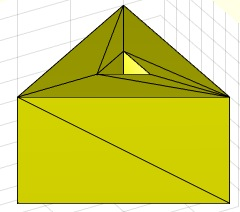% exp_2014_12_13c(n,n2) - Tetrahedron solids based on closed polygon list % processing % (by Tim Lueth, VLFL-Lib, 2014-DEZ-14 as class: EXPERIMENTS)% % dte=exp_2014_12_13c creates first an outside n-point polygon with an % optional m-point polygon insisde, depending on the input parameters. % Then these close polygon lines (CPLs) should be converted into a % tetrahedron triangulation of an extrusion of the CPL with the height z.% For this purpose a frame is created outside of the polygon first. Then % contour by contour a delaunay triangulation is created.% % The concept of this procedure is similar to CLPrecontour but it works % on tetrahedron solids.% While facet based surfaces are enclosed and described by polygons % (cw,ccw)% terahedron based solids are enclosed and described by surfaces.% In this procedure we create tetrahedron by simple 2.5D extrusion of % CPLs and add or remove the inner parts.% % % Created after exp_2014_12_14 the same day later. (Status of: 2014-12-19)% % dte=exp_2014_12_13c([n,n2])% === INPUT PARAMETERS ===% n: Number of points of the outer polygon, default is 4% n2: Optional number of points of the inner polygon, default is 0% === OUTPUT RESULTS ======% dte: resulting tetrahedron triangulation%
###### TRselect(TR,TL)- returns a reduced tetrahedron triangulation for a given tetrahedron list% TRselect(TR,TL) - returns a reduced tetrahedron triangulation for a % given tetrahedron list% (by Tim Lueth, VLFL-Lib, 2014-DEZ-14 as class: TETRAHEDRONS)% % In some cases, for instance when surfaces are tessellated, it makes % more sense to operate using only the required vertices. Afterwards, the % vertex list is much shorter, the index list reindexed. By using % TL=SI(NTL), EL=SI(NEL) for a new edge list or FL=SI(NFL) for a facet % list, the resulting EL or EL are backindexed to the original vertex % list.% TL=SI(NTL); if size(TL,2)==1; TL=TL'; end;% FL=SI(NFL); if size(FL,2)==1; FL=FL'; end;% EL=SI(NEL); if size(EL,2)==1; EL=EL'; end;% This procedure is similar to VLFLselect or VLELselect.% (Status of: 2014-12-14)% % [TR,SI,TL]=TRselect(TR,TL)% === INPUT PARAMETERS ===% TR: Tetrahedron triangulation% TL: Subset of TR.ConnectivityList% === OUTPUT RESULTS ======% TR: New tetrahedron triangulation consisting of minimal points for the % ConnectivityList subset% SI: Sorting index% TL: Reindexed subset of TR.ConnectivityList%
###### TRcat(TRA,TRB)- returns a concatenation of tetrahedron solids without any checks% TRcat(TRA,TRB) - returns a concatenation of tetrahedron solids without % any checks% (by Tim Lueth, VLFL-Lib, 2014-DEZ-14 as class: TETRAHEDRONS)% % Use TRunique afterwards if desired, to remove doubled points (Status % of: 2014-12-14)% % TR=TRcat(TRA,TRB)% === INPUT PARAMETERS ===% TRA: Triangulation A% TRB: Triangulation B% === OUTPUT RESULTS ======% TR: Concatenation of Triangulation of A and B%
###### exp_2014_12_13b(n,n2)- Tetrahedron solids based on closed polygon list processing% exp_2014_12_13b(n,n2) - Tetrahedron solids based on closed polygon list % processing % (by Tim Lueth, VLFL-Lib, 2014-DEZ-13 as class: EXPERIMENTS)% % The concept of this procedure is similar to CLPrecontour but it works % on tetrahedron solids.% While facet based surfaces are enclosed and described by polygons % (cw,ccw)% terahedron based solids are enclosed and described by surfaces.% In this procedure we create tetrahedron by simple 2.5D extrusion of % CPLs and add or remove the inner parts.% % % Created after exp_2014_12_14 the same day later. (Status of: 2014-12-13)% % dtx=exp_2014_12_13b([n,n2])% === INPUT PARAMETERS ===% n: Number of points of the outer polygon, default is 4% n2: Optional number of points of the inner polygon, default is 0% === OUTPUT RESULTS ======% dtx: resulting tetrahedron triangulation%
###### TRunique(TR)- returns a tetrahedron triangulation with unique Points% TRunique(TR) - returns a tetrahedron triangulation with unique Points% (by Tim Lueth, VLFL-Lib, 2014-DEZ-13 as class: TETRAHEDRONS)% % Reduces the Points to unique Points and reindexes the Connectivity List % (Status of: 2014-12-13)% % TR=TRunique(TR)% === INPUT PARAMETERS ===% TR: Tetrahedron triangulation% === OUTPUT RESULTS ======% TR: Tetrahedron triangulation with unique Points%
###### TRorder(TR)- reorders the tetrahedrons to individual solids% TRorder(TR) - reorders the tetrahedrons to individual solids% (by Tim Lueth, VLFL-Lib, 2014-DEZ-13 as class: TETRAHEDRONS)% % All tetrahedrons of a solid use the same points. In case that a TR % (triangulation) has a ConnectivityList that in fact is separated into % several solids, this fnctns reorders the ConnectivityList to connected % tetrahedrons and returns also a list with starting tetrahedron and end % tetrahedron of the list. (Status of: 2017-02-21)% % See also: ELorder, FLorder, FLseparate, SGorder, SGseparate% % [TR,SIL]=TRorder(TR)% === INPUT PARAMETERS ===% TR: tetrahedron triangulation consisting of several solids% === OUTPUT RESULTS ======% TR: tetrahedron triangulation consisting of several separated solids% SIL: Solid index list (start and end-tetrahedron) in ConnectivityList%
###### exp_2014_12_13a (n,n2)- Tetrahedron solids based on closed polygon list processing% exp_2014_12_13a (n,n2) - Tetrahedron solids based on closed polygon % list processing % (by Tim Lueth, VLFL-Lib, 2014-DEZ-13 as class: EXPERIMENTS)% % The concept of this procedure is similar to CLPrecontour but it works % on tetrahedron solids.% While facet based surfaces are enclosed and described by polygons % (cw,ccw)% terahedron based solids are enclosed and described by surfaces.% In this procedure we create tetrahedron by simple 2.5D extrusion of % CPLs and add or remove the inner parts.% % % Created after exp_2014_12_14 the same day later. (Status of: 2014-12-13)% % exp_2014_12_13a([n,n2])% === INPUT PARAMETERS ===% n: Number of points of the outer polygon, default is 4% n2: Optional number of points of the inner polygon, default is 0%
###### exp_2014_12_14(n,n2)- Experiment to show the structure of Delaunay Tesselation of Tetrahedron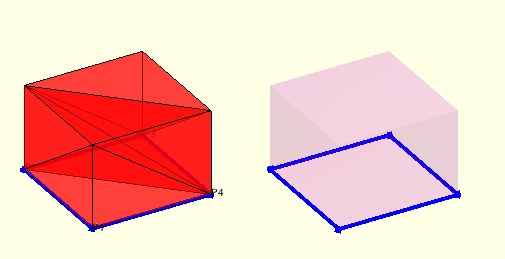% exp_2014_12_14(n,n2) - Experiment to show the structure of Delaunay % Tesselation of Tetrahedron% (by Tim Lueth, VLFL-Lib, 2014-DEZ-13 as class: EXPERIMENTS)% % TR=exp_2014_12_14([n,n2])% === INPUT PARAMETERS ===% n: Number of edges of an out circle% n2: Optional number of edges of an inner circle; default 0% === OUTPUT RESULTS ======% TR: % % EXAMPLE: exp_2014_12_14 (10,4)%
###### exp_2014_12_13- PUBLISHABLE EXPERIMENT shows the difference between triangulation and delaunayTriangulation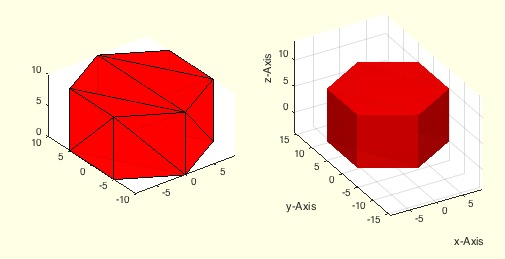% exp_2014_12_13 - PUBLISHABLE EXPERIMENT shows the difference between % triangulation and delaunayTriangulation% (by Tim Lueth, VLFL-Lib, 2014-DEZ-12 as class: EXPERIMENTS)% % This procedure shows the difference between triangulation and % delaunayTriangulation (Status of: 2014-12-13)% % exp_2014_12_13%
###### exp_2014_12_12- PUBLISHABLE EXPERIMENT shows the difference between triangulation and delaunayTriangulation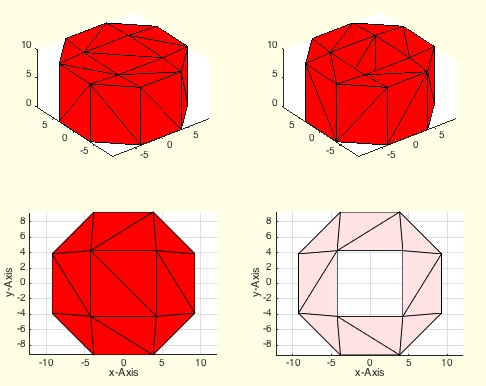% exp_2014_12_12 - PUBLISHABLE EXPERIMENT shows the difference between % triangulation and delaunayTriangulation% (by Tim Lueth, VLFL-Lib, 2014-DEZ-11 as class: EXPERIMENTS)% % This procedure shows the difference between triangulation and % delaunayTriangulation (Status of: 2014-12-12)% % exp_2014_12_12%
###### exp_2014_12_11- PUBLISHABLE EXPERIMENT for tetrahedron generation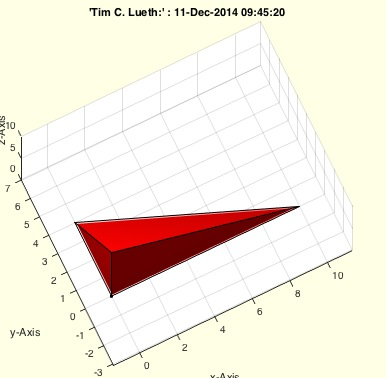% exp_2014_12_11 - PUBLISHABLE EXPERIMENT for tetrahedron generation% (by Tim Lueth, VLFL-Lib, 2014-DEZ-10 as class: EXPERIMENTS)% % exp_2014_12_11%
###### exp_2014_12_10(XFL)- EXPERIMENT just shows for a prismatic object based on a traingle the evolving tetrahedron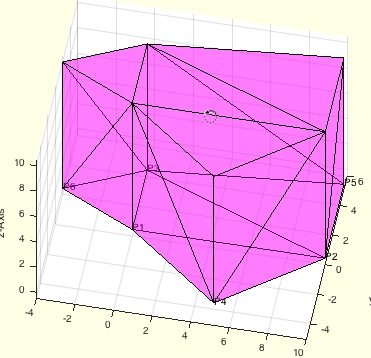% exp_2014_12_10(XFL) - EXPERIMENT just shows for a prismatic object % based on a traingle the evolving tetrahedron% (by Tim Lueth, VLFL-Lib, 2014-DEZ-10 as class: EXPERIMENTS)% % Palma Cala Major - Using % TL=TLofFL([3 1 2],size(PL,1)) and% FL=FLofTetrahedron(TL) (Status of: 2014-12-10)% % exp_2014_12_10([XFL])% === INPUT PARAMETERS ===% XFL: Facet for the triangle; default is [3 1 2]% % EXAMPLE: Just try% exp_2014_12_10%
###### VLTLplot(VL,TL,c,so)- plots a tetrahedron list as one volume or as separated tetrahedrons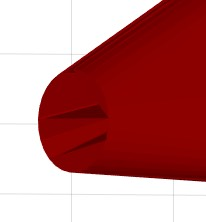% VLTLplot(VL,TL,c,so) - plots a tetrahedron list as one volume or as separated tetrahedrons% (by Tim Lueth, VLFL-Lib, 2014-DEZ-09 as class: TETRAHEDRONS)% % uses tetramesh to print all tetrahedrons in one step.% Each tetrahedron is plotted seperately (slow) (Status of: 2017-02-08)% % See also: VLFLplot, SGplot, TRplot, VLFLplot4% % h=VLTLplot(VL,TL,[c,so])% === INPUT PARAMETERS ===% VL: Vertex list% TL: Tetrahedrons list% c: color% so: true = single handle, false = separated handles% === OUTPUT RESULTS ======% h: handles to graphics object%
###### exp_2014_12_09- just shows for a prismatic object based on a traingle the tetrahedron% exp_2014_12_09 - just shows for a prismatic object based on a traingle % the tetrahedron% (by Tim Lueth, VLFL-Lib, 2014-DEZ-09 as class: EXPERIMENTS)% % Palma Cala Major (Status of: 2014-12-09)% % exp_2014_12_09%
###### exp_2014_12_08(XFL)- EXPERIMENT just shows for a prismatic object based on a traingle the evolving tetrahedron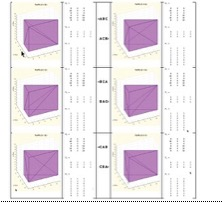% exp_2014_12_08(XFL) - EXPERIMENT just shows for a prismatic object % based on a traingle the evolving tetrahedron% (by Tim Lueth, VLFL-Lib, 2014-DEZ-08 as class: EXPERIMENTS)% % Palma Cala Major - Using % TL=TLofFL([3 1 2],size(PL,1)) and% FL=FLofTetrahedron(TL) (Status of: 2014-12-10)% % exp_2014_12_08([XFL])% === INPUT PARAMETERS ===% XFL: Facet for the triangle; default is [3 1 2]% % EXAMPLE: Just try% exp_2014_12_08 ([ 1 2 3])%
###### exp_2014_12_07(CPL);- EXPERIMENT Extrude a CPL into a tetrahedron representation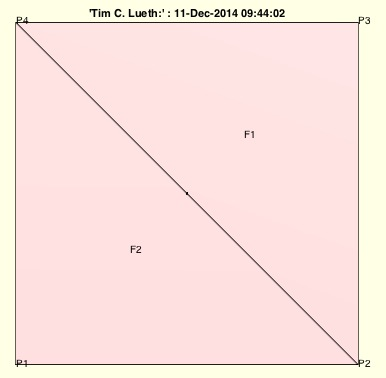% exp_2014_12_07(CPL); - EXPERIMENT Extrude a CPL into a tetrahedron % representation% (by Tim Lueth, VLFL-Lib, 2014-DEZ-06 as class: EXPERIMENTS)% % Work in progress, Should use the slicing result of exp_2014_12_06% Not finished (Status of: 2014-12-10)% % [PL,FL]=exp_2014_12_07([CPL]);% === INPUT PARAMETERS ===% CPL): Closed polygon list% === OUTPUT RESULTS ======% PL: Point list% FL: Facet list%
###### TLofFL(FL,n)- converts a planar facet list into a 2.5 tetrahedron list% TLofFL(FL,n) - converts a planar facet list into a 2.5 tetrahedron list% (by Tim Lueth, VLFL-Lib, 2014-DEZ-06 as class: TETRAHEDRONS)% % Work in progress, does not work correctly for more than 1 triangle % (Status of: 2014-12-06)% % TL=TLofFL(FL,[n])% === INPUT PARAMETERS ===% FL: Facet list% n: number of points in vertex list% === OUTPUT RESULTS ======% TL: Tetrahedron list%
###### FLshift(FL)- returns a shifted and ordered facet list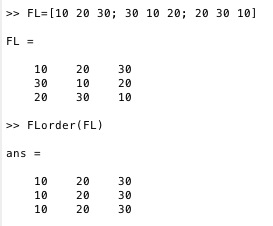% FLshift(FL) - returns a shifted and ordered facet list% (by Tim Lueth, VLFL-Lib, 2014-DEZ-06 as class: AUXILIARY PROCEDURES)% % The facet list is rotated line by line so that the smallest vertex % index is in the left column.% % In contrast to FLorder, the rows are not sorted afterwards. (Status of: % 2015-08-05)% % FL=FLshift(FL)% === INPUT PARAMETERS ===% FL: Original facet list% === OUTPUT RESULTS ======% FL: Final facet list% % EXAMPLE: % FL=[10 20 30; 30 10 20; 20 30 10] % FL=[FL*1.5;FL]% FLshift(FL)%
###### TRplot(TR,c,fb)- plots a tetrahedron triangulation similar to SGplot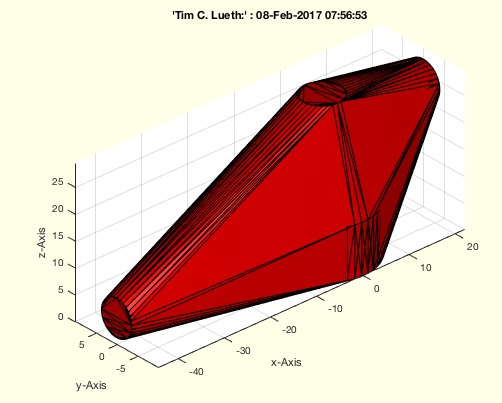% TRplot(TR,c,fb) - plots a tetrahedron triangulation similar to SGplot% (by Tim Lueth, VLFL-Lib, 2014-DEZ-06 as class: TETRAHEDRONS)% % more or less: tetramesh(TR4)% % (Status of: 2017-02-08)% % See also: VLFLplot, SGplot, VLTLplot, VLFLplot4% % h=TRplot(TR,[c,fb])% === INPUT PARAMETERS ===% TR: Tetrahedron% c: color% fb: true=freeBoundary [default], false=tetrahedrons,% === OUTPUT RESULTS ======% h: handles% % EXAMPLE: Show the convex hull% A=SGsample(17)% TR=delaunayTriangulation(A.VL);% TRplot(TR)%
###### TRofCPLz (CPL,z)- returns a valid tetrahedron representation of a solid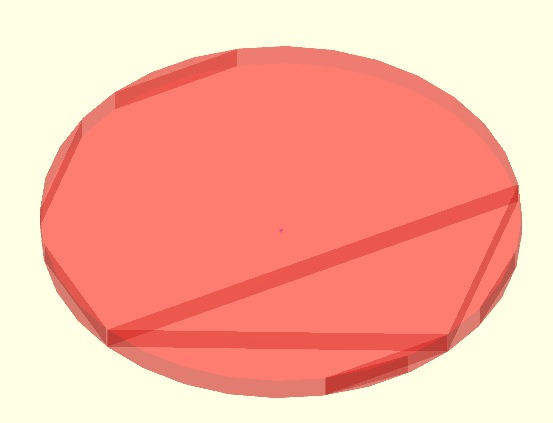% TRofCPLz (CPL,z) - returns a valid tetrahedron representation of a solid% (by Tim Lueth, VLFL-Lib, 2014-DEZ-06 as class: TETRAHEDRONS)% % This procedures is an extension of the first ideas with DTofPLELz % (2014-01-14). This time the appraoch is based on the concepts Closed % Polygon Lines (CPL) and Triangulation (TR) wich are both available in % Matlab 2014b. (Status of: 2014-12-06)% % TRofCPLz(CPL,z)% === INPUT PARAMETERS ===% CPL: Closed polygon list for base plate% z: solid height in z-direction%
###### CPLnonmanifold(CPL)- true if a point is used twice in the CPL or PL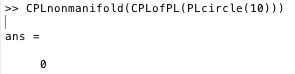% CPLnonmanifold(CPL) - true if a point is used twice in the CPL or PL% (by Tim Lueth, VLFL-Lib, 2014-DEZ-06 as class: AUXILIARY PROCEDURES)% % Slow, try to avoid if possbile (Status of: 2014-12-06)% % nm=CPLnonmanifold(CPL)% === INPUT PARAMETERS ===% CPL: closed polygon list% === OUTPUT RESULTS ======% nm: true if non manifold points% % EXAMPLE: % CPLnonmanifold(CPLofPL(PLcircle(10)))%
###### CPLofPL(PL)- appends the first point to a point list of required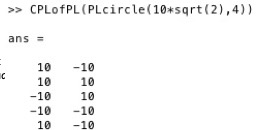% CPLofPL(PL) - appends the first point to a point list of required% (by Tim Lueth, VLFL-Lib, 2014-DEZ-06 as class: AUXILIARY PROCEDURES)% % The last point of a CPL has to be the first point.% Slow, please try to avoid if possibile (Status of: 2014-12-26)% % See also: PLofCPL% % CPL=CPLofPL(PL)% === INPUT PARAMETERS ===% PL: point list% === OUTPUT RESULTS ======% CPL: closed polygon list%
###### exp_2014_12_06(z)- PUBLISHABLE experiment to show the result of different slicing techniques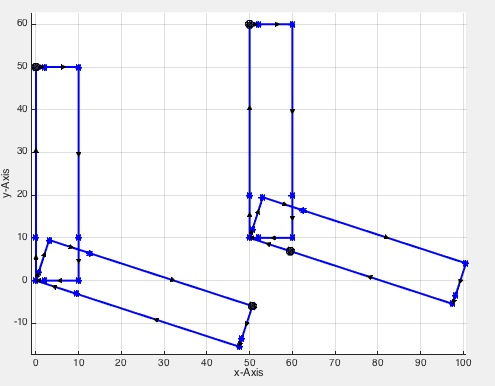% exp_2014_12_06(z) - PUBLISHABLE experiment to show the result of % different slicing techniques% (by Tim Lueth, VLFL-Lib, 2014-DEZ-06 as class: EXPERIMENTS)% % Does work currently only with correct sample files (no errors in the % VL/FL) (Status of: 2014-12-06)% % CPL=exp_2014_12_06([z])% === INPUT PARAMETERS ===% z: z-coordinate for slicing plane% === OUTPUT RESULTS ======% CPL: Resulting contour polygon list%
###### CPLrecontour(CPL,frm)- returns a contour list for a sliced/cutted object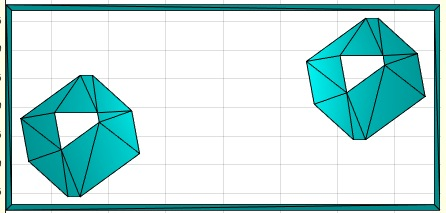% CPLrecontour(CPL,frm) - returns a contour list for a sliced/cutted % object% (by Tim Lueth, VLFL-Lib, 2014-DEZ-05 as class: ANALYTICAL GEOMETRY)% % A given CPL (closed polygon list, contains NaN) is ordered by x and y % values first. Then each polygon is analyzed regarding the clockwise % direction (cw/ccw). Then all polygons are united or subtracted % depending on their direction. To have a defined start point, a frame is % generated that is the maximum outside border of the polygons inside. By % a second parameter it is possible to delete the frame from the % resulting CPL.% The resulting list is a always VALID closed polygon list.% % (Status of: 2014-12-06)% % NPL=CPLrecontour(CPL,[frm])% === INPUT PARAMETERS ===% CPL: Closed polygon list, contains NaN% frm: true=frame, false=no frame% === OUTPUT RESULTS ======% NPL: resulting closed polygon list (with our without a frame)%
###### exp_2014_12_04- PUBLISHABLE EXPERIMENT SIMILAR TO 2014_12_05 but for STL Files% exp_2014_12_04 - PUBLISHABLE EXPERIMENT SIMILAR TO 2014_12_05 but for % STL Files% (by Tim Lueth, VLFL-Lib, 2014-DEZ-05 as class: EXPERIMENTS)% % Work in progress% This experiment was used to develop the CPLrecontour procedure an to % see the effect of attached edges, non manifold points and non manifold % edges. By reading in different STL files is becomes obvious that % corrupted STL files are a serious problem for any slicer. (Status of: % 2014-12-10)% % exp_2014_12_04%
###### CPLremstraight(CPL)- removes points from straight lines in all contours of CPL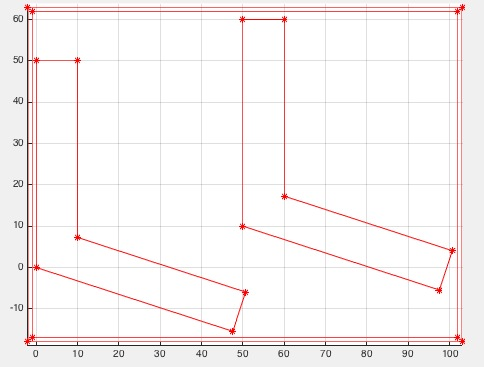% CPLremstraight(CPL) - removes points from straight lines in all contours of CPL% (by Tim Lueth, VLFL-Lib, 2014-DEZ-05 as class: AUXILIARY PROCEDURES)% % =======================================================================% OBSOLETE (2017-07-13) - USE FAMILY 'CVLremstraight' INSTEAD% =======================================================================% % Splits non-manifold points(not edges), shift the contours to start with % minx/miny, order from left bottum to right up AND remove points that % are on a straight line. (Status of: 2014-12-26)% % Introduced first in SolidGeometry 2.0% % See also: [ CVLremstraight ] ; VLremstraightCVL% % NPL=CPLremstraight(CPL)% === INPUT PARAMETERS ===% CPL: Original closed polygon list (includes NaN)% === OUTPUT RESULTS ======% NPL: CPL list: Splitted, shifted, and ordered list with only two points % for a straight line% % See also: [ CVLremstraight ] ; VLremstraightCVL%
###### CPLorder(CPL,smax)- returns an xy ordered and shifted CPL% CPLorder(CPL,smax) - returns an xy ordered and shifted CPL% (by Tim Lueth, VLFL-Lib, 2014-DEZ-05 as class: AUXILIARY PROCEDURES)% % All polygons are now orderd from outside to inside, so that they can % processed one after another.% They ordered from minx/miny to maxx/maxy or in the opposite way. % (Status of: 2015-08-16)% % NCPL=CPLorder(CPL,[smax])% === INPUT PARAMETERS ===% CPL: Closed Polygon List (including NaN)% smax: max if true, min if false; default is false% === OUTPUT RESULTS ======% NCPL: Ordered closed polygon list%
###### CPLcircshift(PL,cl)- shifts & closes a PL. First point is [minx miny]% CPLcircshift(PL,cl) - shifts & closes a PL. First point is [minx miny] % (by Tim Lueth, VLFL-Lib, 2014-DEZ-05 as class: AUXILIARY PROCEDURES)% % Attention the length of the NPL can be increased to add the first point % as last point.% cl = false => CPL may be longer than PL% CL = true => CPL is PL if the input argument was PL (Status of: % 2014-12-26)% % NPL=CPLcircshift(PL,[cl])% === INPUT PARAMETERS ===% PL: Original PL or CPL% cl: constant legnth; default is false% === OUTPUT RESULTS ======% NPL: Shifted and CPL%
###### CPLsplitpoints(CPL)- Splits non manifold points in a CPL% CPLsplitpoints(CPL) - Splits non manifold points in a CPL% (by Tim Lueth, VLFL-Lib, 2014-DEZ-05 as class: AUXILIARY PROCEDURES)% % Sometimes as a result of slicing process, there are non manifold points % (joint points) of two closed CPLs. This is detected and corrected by % this procedure. If there are - after a slicing procedure - non manifold % edges in a plane, somebody made a mistake during the design. Non % manifold points in a plane can be resolved. Non manifold edge not by % this procedure. % Intellectual property: This can be used as copy protection for 3D print % protection. It is possible visualize but not possible to print. (Status % of: 2017-04-23)% % See also: PLsplitpointsofCPL% % NPL=CPLsplitpoints(CPL)% === INPUT PARAMETERS ===% CPL: Contour polygon list% === OUTPUT RESULTS ======% NPL: Corrected Contour polygon list%
###### CPLisccw(CPL)- returns index list for CPL direction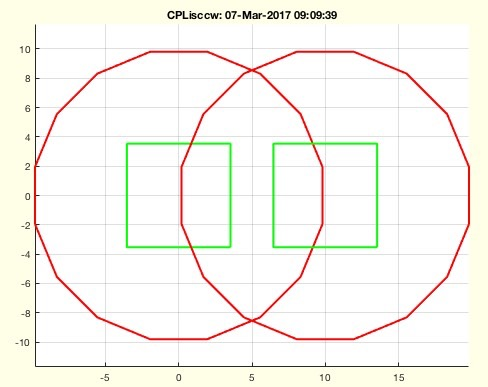% CPLisccw(CPL) - returns index list for CPL direction% (by Tim Lueth, VLFL-Lib, 2014-DEZ-05 as class: ANALYTICAL GEOMETRY)% % the same as ~ispolycw (Status of: 2017-05-27)% % See also: CPLisccwinout, CPLsortinout, CPLisccwcorrected% % [cwi,nani]=CPLisccw(CPL)% === INPUT PARAMETERS ===% CPL: CPL% === OUTPUT RESULTS ======% cwi: counter-clockwise index% nani: NAN-indices% % EXAMPLE: % CPLisccw(CPLsample(11))%
###### exp_2014_12_05- PUBLISHABLE EXPERIMENT for slicer reconstruction, analyzing non manifold problems% exp_2014_12_05 - PUBLISHABLE EXPERIMENT for slicer reconstruction, % analyzing non manifold problems% (by Tim Lueth, VLFL-Lib, 2014-DEZ-05 as class: EXPERIMENTS)% % Work in progress% This experiment was used to develop the CPLrecontour procedure an to % see the effect of attached edges, non manifold points and non manifold % edges. (Status of: 2014-12-05)% % exp_2014_12_05%
###### SGtrans(SG,T)- multi transformation modes for a solid geometry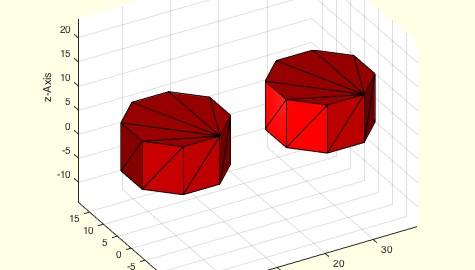% SGtrans(SG,T) - multi transformation modes for a solid geometry% (by Tim Lueth, VLFL-Lib, 2014-DEZ-03 as class: ANALYTICAL GEOMETRY)% % Does currently not support a list of solids.% Combines SGytrans0, SGtrans1, SGtransP, SGtransR, SGtransT depending on % the transformation value% SGtrans(SG,0)% SGtrans(SG,1)% SGtrans(SG,[0 0 10]% SGtrans(SG,[0; 0 ;10])% SGtrans(SG,rot(pi/3))% SGtrans(SG,rot(pi/3,pi/2,0))% SGtrans(SG,TofDPhiH(20,pi/6,10)) (Status of: 2014-12-03)% % [SG1,varargout]=SGtrans(SG,T)% === INPUT PARAMETERS ===% SG: Solid Geometry% T: Transformation Value, Vector, or Matrix% === OUTPUT RESULTS ======% SG1: Transforms solid geometry% varargout: %
###### SGsample(Nr,T)- returns a solid geometry for sample bodies used for testing and experiments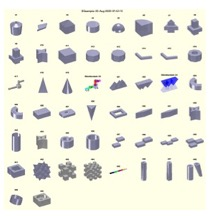% SGsample(Nr,T) - returns a solid geometry for sample bodies used for testing and experiments% (by Tim Lueth, VLFL-Lib, 2014-DEZ-03 as class: MODELING PROCEDURES)% % there are different solids in different structures that support the % test of different geometries (Status of: 2017-04-23)% % See also: CPLsample, VLsample, PLsample% % SG=SGsample([Nr,T])% === INPUT PARAMETERS ===% Nr: Nr of sample solid; default is show% T: Parameter for SGtrans (Value, Vector, Matrix)% === OUTPUT RESULTS ======% SG: Solid geometry%
###### exp_2014_12_03- PUBLISHABLE EXPERIMENT for VLFL reconstuction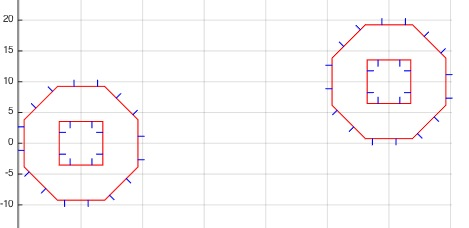% exp_2014_12_03 - PUBLISHABLE EXPERIMENT for VLFL reconstuction% (by Tim Lueth, VLFL-Lib, 2014-DEZ-03 as class: EXPERIMENTS)% % Work in progress% Uses SGsample(12,13,14,15) to generate a solid, slice it a cutting % plane and calculate the evolving contour (Status of: 2014-12-05)% % exp_2014_12_03%
###### -###### PLrand(n,r,c)- returns a point list with random points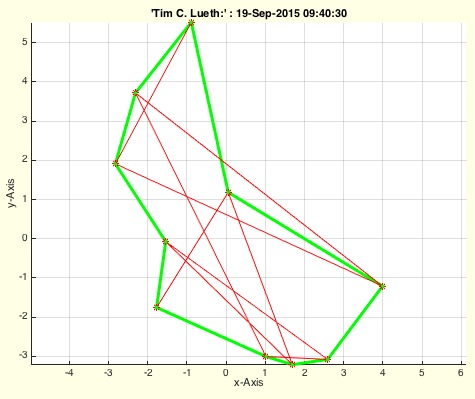% PLrand(n,r,c) - returns a point list with random points% (by Tim Lueth, VLFL-Lib, 2014-DEZ-02 as class: AUXILIARY PROCEDURES)% % mainly r*rand(n,2) but combined with PLsortC (Status of: 2017-01-05)% % See also: PLcircle, PLcircseg, PLevolvente, PLgear, PLhelix, PLkidney, % PLspiral, PLsquare, PLstar% % [PL,CPL]=PLrand([n,r,c])% === INPUT PARAMETERS ===% n: number of points; default is 10% r: Radius; default is 10% c: Center; default is [0 0]% === OUTPUT RESULTS ======% PL: Point list nx2 (unsorted)% CPL: Point list nx2 (sorted as contour), not closed% % EXAMPLE: close all;% CPLplot(PLsortC(PLrand(20,10))); view(0,90); axis equal;%
###### PLsortC(PL,d)- returns a point list that is sorted around its' center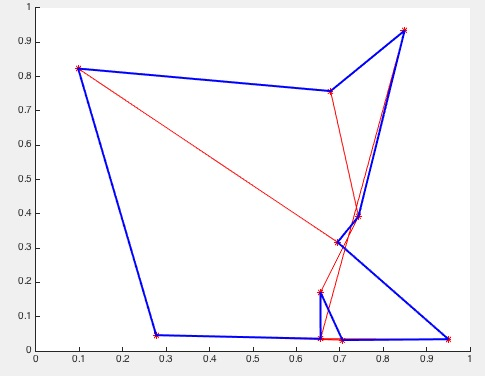% PLsortC(PL,d) - returns a point list that is sorted around its' center% (by Tim Lueth, VLFL-Lib, 2014-DEZ-02 as class: AUXILIARY PROCEDURES)% % Uses VLsortC. All points are part of the resulting list. (Status of: % 2014-12-02)% % [PL,WL,DL]=PLsortC(PL,[d])% === INPUT PARAMETERS ===% PL: Original point list% d: order same angle: 'outer' | 'inner'; default is 'inner'% === OUTPUT RESULTS ======% PL: Sorted point list% WL: Angle list% DL: Distance list% % EXAMPLE: Sort a random point list% close all; % for i=1:10; PL(i,:)=[rand rand]; end; % CPLplot (PL); CPLplot(PLsortC(PL),'b',2); show%
###### SGswap(SG)- returns a SG with inverted facets% SGswap(SG) - returns a SG with inverted facets% (by Tim Lueth, VLFL-Lib, 2014-NOV-30 as class: AUXILIARY PROCEDURES)% % similar to FLswap, but for solids (Status of: 2014-11-30)% % SG=SGswap(SG)% === INPUT PARAMETERS ===% SG: Solid geometry% === OUTPUT RESULTS ======% SG: Solid geometry with inverted facets% % EXAMPLE: Generation of a hollow ellipsoid% CPL=PLcircle(30); CPL(:,2)=CPL(:,2)*2; % SG=SGcat(SGofCPLrot(CPL),SGswap(SGofCPLrot(CPL*0.5)));% SGplot(SG); % SGwriteSTL(SG);%
###### SGbool3(flag,A,B,thr,tho)- Boolean operators for solid geometries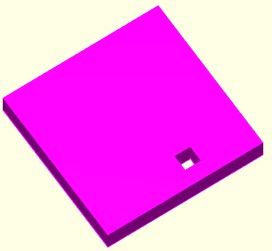% SGbool3(flag,A,B,thr,tho) - Boolean operators for solid geometries% (by Tim Lueth, VLFL-Lib, 2014-NOV-29 as class: SURFACES)% % First usable procedure achieved on 2015-01-10.% It took several weeks to implement it. (Status of: 2015-01-11)% % SGX=SGbool3(flag,A,B,[thr,tho])% === INPUT PARAMETERS ===% flag: Operator such as 'A','B','+','x'% A: Solid A% B: Solid B% thr: grid resolution for A and B; default is 1e-5% tho: grid resolution for the output result; default is 1e-4;% === OUTPUT RESULTS ======% SGX: Final resulting geometry%
###### SGofCPLrot(CPL,n)- returns a solid geometry from of a z-axis rotated closed polygon list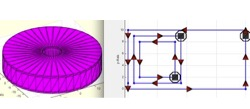% SGofCPLrot(CPL,n) - returns a solid geometry from of a z-axis rotated closed polygon list% (by Tim Lueth, VLFL-Lib, 2014-NOV-29 as class: MODELING PROCEDURES)% % Basic modeling procedure such as SGofCPLz. An important feature of this % procedure is the insertion on contour points that make it possible to % separate the object easily in z. Nevertheless, if case that embeded % circles are rotated a remarkable number of new points are inserted at % the outside contour. (Status of: 2017-03-19)% % See also: SGofCPLsphere, SGbeating, SGofCPLz, SGofCPLT% % SG=SGofCPLrot(CPL,[n])% === INPUT PARAMETERS ===% CPL: Radius-Z List% n: optional number of edges of the rotated polygon% === OUTPUT RESULTS ======% SG: Solid Geometry (SG.VL, SG.FL, SG.CPL)% % EXAMPLE: CPL=[0 0; 20 0; 20 10; 0 10; NaN NaN; 1 1; 9 1; 9 9; 1 9; NaN % NaN; 2 2; 8 2; 8 8; 2 8 ];% CPL(:,1)=CPL(:,1)+5% CPL=PLcircle(10);% CPL=[0 10; 2 8; 0 5; 0 4; 4 1; 0 0];% A=SGofCPLrot (CPL); SGchecker(A); % VLFLviewer(A); VLFLplotlight (1,0.5), view(-30,30); % figure(2); PLELofCPL(A.CPL);%
###### VLFLofCPLrot(CPL,n)- returns a solid geometry from of a radius-z list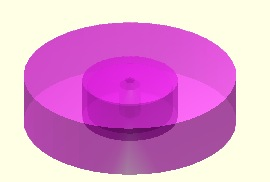% VLFLofCPLrot(CPL,n) - returns a solid geometry from of a radius-z list % (by Tim Lueth, VLFL-Lib, 2014-NOV-29 as class: MODELING PROCEDURES)% % [VL,FL,CPL]=VLFLofCPLrot(CPL,[n])% === INPUT PARAMETERS ===% CPL: Radius-Z List% n: number of edges of the prismatic cross section% === OUTPUT RESULTS ======% VL: Vertex list% FL: Facet list% CPL: Resulting contour polygon list% % EXAMPLE: Generating different solids by rotating a contour% CPL=[0 0; 20 0; 20 10; 0 10; NaN NaN; 1 1; 9 1; 9 9; 1 9; NaN NaN; 2 2; % 8 2; 8 8; 2 8 ];% CPL(:,1)=CPL(:,1)+5% CPL=PLcircle(10);% CPL=[0 10; 2 8; 0 5; 0 4; 4 1; 0 0];% [VL,FL,NCPL]=VLFLofCPLrot (CPL); % VLFLchecker(VL,FL); % VLFLviewer(VL,FL); VLFLplotlight (1,0.5), view(-30,30); PLELofCPL % (NCPL); show%
###### CPLuniteCPL(CPL)- returns a correct directed closed polygon list from a undirected closed polygon list% CPLuniteCPL(CPL) - returns a correct directed closed polygon list from % a undirected closed polygon list% (by Tim Lueth, VLFL-Lib, 2014-NOV-27 as class: ANALYTICAL GEOMETRY)% % This procedure returns a corrects ordered (cw/ccw) CPL.% To get the right order (ccw/cw) for a CPL 3D extrusion, use flip(CPL) % before.% A feature is that there are additional points on straight lines, that % help to separate the contour in y-axis into slices (Status of: % 2016-01-06)% % See also: CPLunite, CPLuniteCPL, CPLunitePLFL% % CPL=CPLuniteCPL(CPL)% === INPUT PARAMETERS ===% CPL: Closed polygon list (unidirected)% === OUTPUT RESULTS ======% CPL: Closed polygon list (correctly) directed cw/ccw% % EXAMPLE: Show how the order of polygons can be corrected% CPL=[0 0; 20 0; 20 10; 0 10; NaN NaN; 1 1; 9 1; 9 9; 1 9; NaN NaN; 2 2; % 8 2; 8 8; 2 8 ]% CPL=CPLuniteCPL(CPL);% CPL=flip(CPL);% PLELofCPL(CPL);%